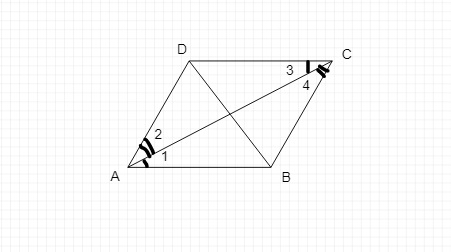# Prove that a diagonal of a Parallelogram divides it into two congruent triangles.

Given :

A  diagonal of a Parallelogram divides it into two congruent triangles.

To do :

We have to prove that a  diagonal of a Parallelogram divides it into two congruent triangles.

Solution :

Take a parallelogram ABCD and join two of its non-adjacent vertices, say A and C.In the parallelogram ABCD, BC || AD and AB || DC.

AC is the transversal between the parallel lines BC and AD, and it is also a transversal between the parallel lines AB and DC.

Let the angles formed by these parallel lines and transversal be angles 1, 2, 3 and 4.

Consider ∆ ABC and ∆ CDA,

∠ 1 = ∠ 3         (since alternate angles are equal)

∠ 2 = ∠ 4         (since alternate angles are equal)

AC = CA          (common side)

So, by the ASA rule ∆ ABC ≅ ∆ CDA.

Therefore, diagonal AC divides parallelogram ABCD into two congruent triangles ABC and CDA.

Hence proved.

Updated on: 10-Oct-2022

675 Views